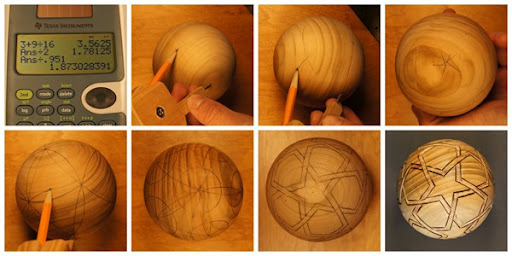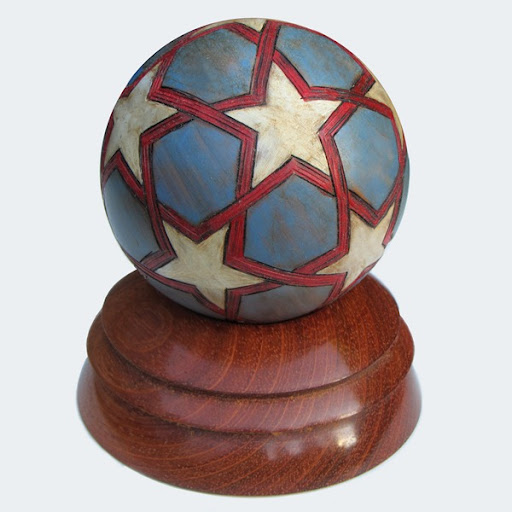### Stars and stripes ball

If you’ve ever been given the task of putting a star design on a sphere, you probably first thought of an icosahedron (or perhaps its dual, the dodecahedron).  Since five triangles meet at each vertex, the icosahedron is intimately linked with pentagons, stars, and the Golden Ratio.

But how do you find the side length, a, of an icosahedron when all you know is the diameter of the circumscribed sphere?  A quick trick is to use Wikipedia, which tells us r = 0.951a.In my case, the sphere’s diameter was 3 9/16 inches, so I used the equation above to find a = 1.873 in.  Now set the compass to this length and get ready for some circular reasoning.

This was a project of my dad’s so he took over and dressed it up real patriotic-like.1.